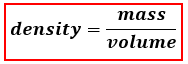# Problem: The density of a substance if 3.400 g/mL. In SI units, the density is:A. 3400 kg/m 3B. 0.3400 kg/m 3C. 3.4 kg/cm 3D. 3.4 kg/m 3E. 3.4 kg/L3

###### FREE Expert Solution
99% (141 ratings)
###### FREE Expert Solution

We’re being asked to determine the density of a substance in SI units. Let’s first determine the SI units for density. When calculating density, we use the following equation:SI units:

99% (141 ratings)###### Problem Details

The density of a substance if 3.400 g/mL. In SI units, the density is:

A. 3400 kg/m 3

B. 0.3400 kg/m 3

C. 3.4 kg/cm 3

D. 3.4 kg/m 3

E. 3.4 kg/L3